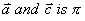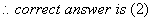Wednesday 17th August 2022
 All India Engineering / Architecture Entrance Examination (AIEEE) CBSE Guess > AIEEE > AIEEE Papers > 2008 > Mathematics AIEEE 2008 MATHEMATICS Q. 1. The mean of the numbers a, b, 8, 5, 10 is 6 and the variance is 6.80 . Then which one of the following gives possible values a and b? a = 1, b = 6 a = 3, b = 4 a = 0, b = 7 a = 5, b = 2 Sol.Possible values of a and b is given by (2) Q. 2. The vector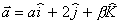lies in the plane of the vectorsand bisects the angle between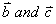. Then which one of the following gives possible values of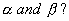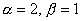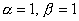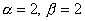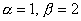Sol.Q. 3.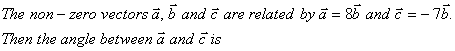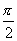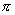0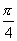Sol. The sign of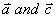are opposite. Hence they are parallel but directions are opposite. Therefore angle between